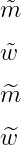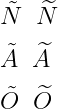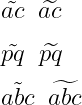# How do you put a tilde(ã, õ, and ñ) over a letter in LaTeX?

There are many uses of tiled symbol. But, the most important thing is to use the tilde symbol over a letter.

In latex, \tilde and \~{} are two important commands for this. And these are the default commands of LaTeX.

Symbol Tilde symbol over a letter
Type Multipurpose
Package No
Commands \tilde, \~{}
Example \tilde{a} → ã
\documentclass{article}
\begin{document}
$\tilde{a}$
$\tilde{n}$
$\tilde{o}$
\end{document}

Output :

ã
ñ
õ


But, \~{} command must be used in text mode. For example

\documentclass{article}
\begin{document}
tilde over a : \~{a} \\
tilde over o : \~{o} \\
tilde over n : \~{n}
\end{document}

Output :

tilde over a : ã
tilde over n : ñ
tilde over o : õ


Notice if you have knowledge of spanish and Portuguese, this tilde symbol is used over special n, a, and o letters. For example

\documentclass{article}
\begin{document}
% use tilde symbol in spanish
ara$\tilde{n}$a \\ [6pt]
ni\~{n}o \\ [6pt]
% use tilde symbol in Portuguese
canç$\tilde{a}$o \\ [6pt]
var\~{o}es
\end{document}

Output :You look at the output above when you pass a character(n, a, and o) in the \tilde command. Then, convert to italic style is not entirely accurate.

However, \~{} command does not change the style of the font. and this is the best practice in the case of language.

## Use responsive tilde symbol over a letter

However, there are some characters that are larger than the size of other characters, so it is best practice to use \widetilde commands instead of \tilde and \~{} commands.

\documentclass{article}
\begin{document}
$\tilde{m}$
$\tilde{w}$
% use widetilde command
$\widetilde{m}$
$\widetilde{w}$
\end{document}

Output :Because look at the output above, the size of the tilde symbol is also constant in the case of \tilde and \~{} commands. However, in the case of the \widetilde command, the tilde symbol is responsibly placed over a letter.

In my opinion, the \widetilde command is best for using tilde symbols on all capital letters.

\documentclass{article}
\begin{document}
$\tilde{N} \;\; \widetilde{N}$
$\tilde{A} \;\; \widetilde{A}$
$\tilde{O} \;\; \widetilde{O}$
\end{document}

Output :However, you must use the \widetilde command for multiple characters. For example

\documentclass{article}
\begin{document}
$\tilde{ac} \;\; \widetilde{ac}$
$\tilde{pq} \;\; \widetilde{pq}$
$\tilde{abc} \;\; \widetilde{abc}$
\end{document}

Output :#### Md Jidan Mondal

LaTeX expert with over 10 years of experience in document preparation and typesetting. Specializes in creating professional documents, reports, and presentations using LaTeX.